While the ideas of gravity and acceleration at and above Earth's surface are taught in first year physics, the acceleration due to gravity below the surface is often ignored or approximated with a homogeneous Earth.  This essay describes a method of finding the gravitational acceleration within a spherical approximation of Earth using a variable density function.

The gravitational acceleration at some distance away from a point mass is given by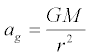where

 ag = Acceleration towards mass M, m·s-2 G = 6.6743×10-11 m3·kg-1·s-2 Universal gravitational constant 1 M = Mass, kg r = Distance from the center of mass, m

The acceleration due to gravity external to a spherically symmetric uniform and continuous distribution of mass can be described by the same equation.

Thus, if we make the assumption that the Earth is a sphere, we can use this equation to find the acceleration due to Earth's gravity for any position on or above the Earth's surface.

But what of the acceleration below the surface?

For a spherically symmetric uniform and continuous distribution of mass, the mass closer to the center of the distribution than the position in question provides a net gravitational acceleration at that position.  The acceleration produced by the shell of mass further from the center, however, sums to zero.  A good tutorial on that fact may be found here.

If we make the assumption that the Earth's density is constant with radiusi.e., that the Earth is homogeneousthen the acceleration falls off in a linear fashion, reaching zero at the center.  That is, if a distribution of mass is spherically symmetric and homogeneous, we could simply calculate the volume of a sphere at radius r and multiply that volume by the density to find the mass.  Since acceleration is proportional to 1/r2 while volume (and hence mass) is proportional to r3, then acceleration is proportional to r within a solid sphere of constant density.

While we will assume that the Earth is spherically symmetric, it is most certainly not homogeneous.  The Earth's density is anything but constant with respect to radius, ranging from 13,000 kg·m-3 at the center to 1,000 kg·m-3 at the surface.  There are large discontinuities in density between the various material layers within the Earth.  Moreover, within each material layer the density can change in a non-linear fashion.

What we need is a function that will provide the mass contained in a sphere of a given radius that allows the density to change with respect to that radius.  If we can calculate the mass, then we can calculate the acceleration due to gravity for that mass.

The solution is to characterize each layer's density as a function of radius, then integrate the density of each layer over radius to find mass.  Since we're assuming the Earth to be spherically symmetric, it'll then be easy to calculate the acceleration due to gravity for any point within the Earth.

The Preliminary Earth Reference Model (PREM) 2 provides a table of densities at different radii within the Earth.  A spreadsheet can be used to generate a quadratic density function to fit the data points for each material layer.  The Excel spreadsheet I used may be found here.

We end up with the following piecewise function for the Earth's density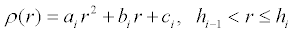where r is the distance from the center of the Earth and where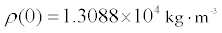and where the indexed constants for each piece of the function are described in the table below.

 i Layer Height hi (m) ai (kg·m-5) bi (kg·m-4) ci (kg·m-3) 1 Inner core 1.2215×106 -2.1773×10-10 1.9110×10-8 1.3088×104 2 Outer core 3.4800×106 -2.4123×10-10 1.3976×10-4 1.2346×104 3 D'' layer 3.6300×106 0 -5.0007×10-4 7.3067×103 4 Lower Mantle 5.7010×106 -3.0922×10-11 -2.4441×10-4 6.7823×103 5 Inner transition zone 1 5.7710×106 0 -2.3286×10-4 5.3197×103 6 Inner transition zone 2 5.9710×106 0 -1.2603×10-3 1.1249×104 7 Outer transition zone 6.1510×106 0 -5.9706×10-4 7.1083×103 8 Low velocity zone & lid 6.3466×106 0 1.0869×10-4 2.6910×103 9 Inner crust 6.3560×106 0 0 2.9000×103 10 Outer crust 6.3680×106 0 0 2.6000×103 11 Ocean 6.3710×106 0 0 1.0200×103

If we plot this function over the radius of the Earth, we obtain the following.

Click on the plot thumbnail for a high resolution image best viewed with a 1280x1024 screen resolution.   The Mathematica source code may be found here.We can find the mass within a sphere of any radius h by integrating the density function ρ(r) in spherical coordinates.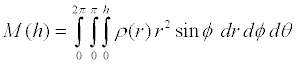If the Earth's density were not a piecewise function, this would be straightforward integration.  However, since the density function contains inconvenient discontinuities, we have to integrate each piece of the function—i.e., each material layer—separately, then sum the masses of each layer appropriately.

As an example, let's derive the equation for the mass of the outer core "below" a height h that lies somewhere within the outer core.  Note that we only want the portion of the outer core's mass below h, but we do not want to include the mass of the inner core since it falls under a different piece of the density function.

We start by setting up the integral of the density function for i = 2 with limits from h1 to h.  Note that h is a variable while h1 is the height of the inner core to outer core boundary given in the table above.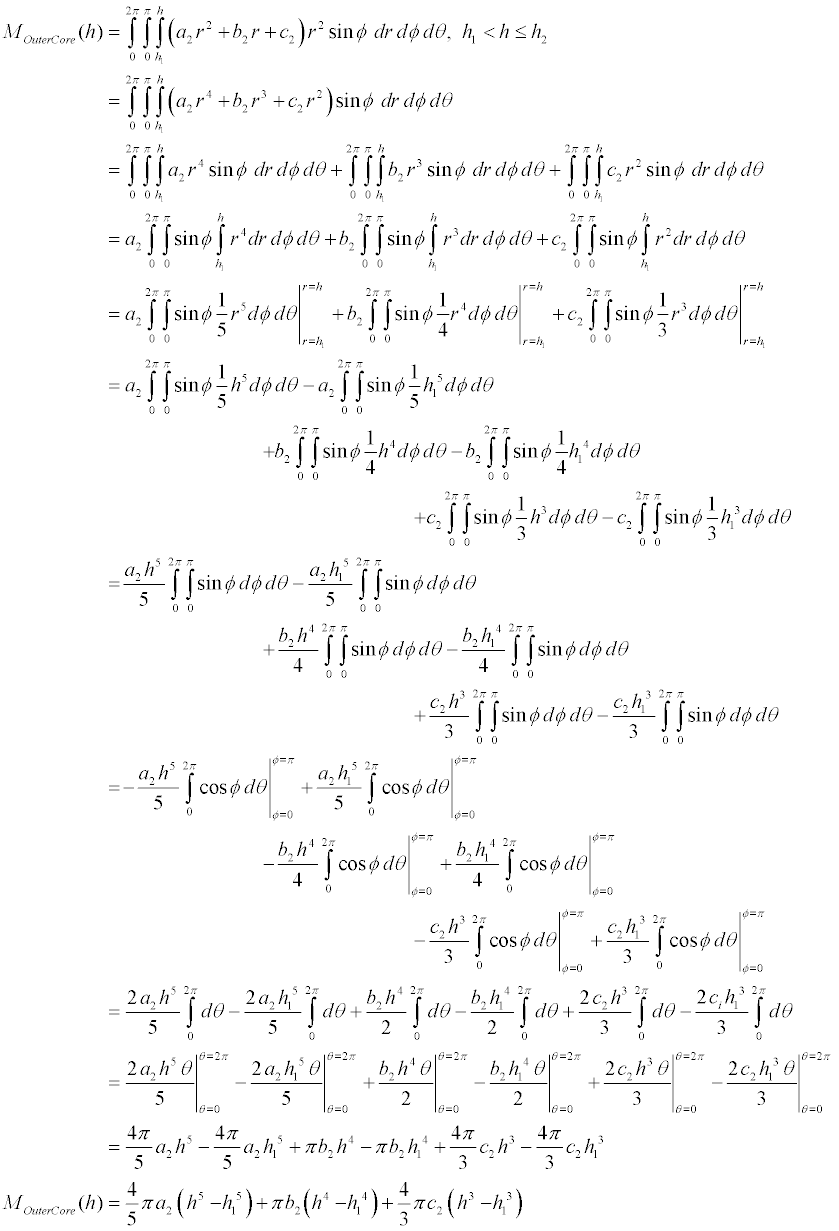where the values of the indexed constants come from the density function table shown above.

The mass functions for the other layers may be similarly derived—but it's fairly obvious that the only difference will be in the indexed constants.

The mass of the entire ith layer is therefore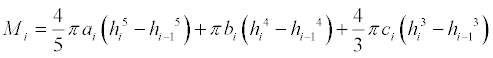where the values of the indexed constants come from the density function table shown above.

To calculate the total mass "below" a given radius r, we sum the masses of the layers that are completely "below" height r, then add the partial mass of the layer that contains radius r.  In the equation below, the height of radius r lies within the nth layer.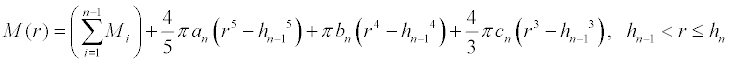where the values of the indexed constants come from the density function table shown above.

Using the volumetric radius of the Earth, 6.3710×106 m, this function evaluates to a total Earth mass of 5.9727×1024 kg.  This is only 0.015% lower than the NASA figure of 5.9736×1024 kg. 3  That's very close given that the PREM densities were inferred from the speed of sound within the Earth using seismographic data.

If we wish to approximate the Earth's internal structure using a constant, average density, we can simply use the volume of a sphere with radius r.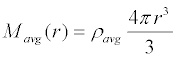which is what that triple integral reduces to when the integrand is a constant, and whereand where rv is the volumetric radius of the Earth.The average density of the Earth evaluates to 5.5139×103 kg·m-3.

Plotting the functions for mass over the radius of the Earth, we obtain the following. The blue line represents the true mass profile, while the dashed gray line represents the average density approximation.

Click on the plot thumbnail for a high resolution image best viewed with a 1280x1024 screen resolution.
The Mathematica source code may be found here.Now that we have a fairly accurate picture of the mass distribution within the Earth, we can return to where we started; that is, to deriving a function for the acceleration due to Earth's gravity that works as well below the surface as it does above.

As discussed above, the gravitational acceleration at some distance away from a point mass or spherically symmetric distribution of mass is given bywhere

 ag = Acceleration towards mass M, m·s-2 G = 6.6743×10-11 m3·kg-1·s-2 Universal gravitational constant 1 M = Mass, kg r = Distance from the center of mass, m

To account for a variable mass and variable density, we substitute our function for mass into the equation for gravitational acceleration.  We also provide a condition for radii outside the Earth.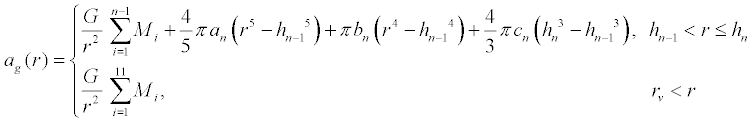where the values of the indexed constants come from the density function table shown above.

In the same fashion, we can also generate a function using the average density..Plotting our function for the radius of the Earth, we obtain the following. The blue line represents the true gravitational acceleration profile, while the dashed gray line represents the gravitational acceleration using the average density approximation.

Click on the plot thumbnail for a high resolution image best viewed with a 1280x1024 screen resolution.
The Mathematica source code may be found here.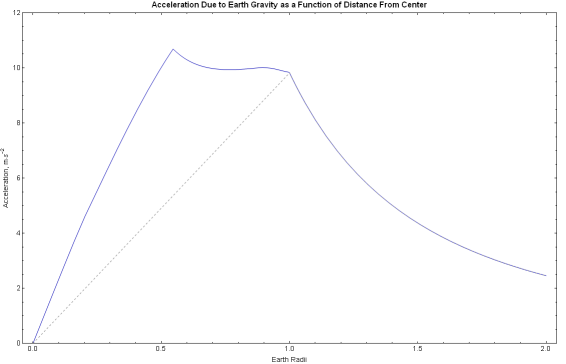The value calculated for gravitational acceleration at Earth's volumetric radius is 9.8212 m·s-2.  According to the World Geodetic System 1984 (WGS84), the true surface acceleration on Earth varies from 9.7803 m·s-2 on the equator to 9.8322 m·s-2 at 90° latitude; the mean value is 9.7976 m·s-2. 4  Thus, our derivation is higher than the mean by 0.24%.  A slight disagreement with true surface acceleration is to be expected since our derivation does not account for centripetal acceleration or the fact that the Earth is an oblate spheroid, not a sphere.  Both phenomena are caused by the Earth's rotation.

For reference, the WGS84 gravity formula, which takes these phenomena into account, is: 4where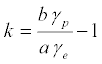where

 γ(f) = Net surface acceleration, m·s-2 f = Geodetic latitude (map latitude) γe = 9.7803253359 m·s-2 Surface acceleration at the equator γp = 9.8321849378 m·s-2 Surface acceleration at the poles a = 6378137.0 m Semi-major axis of the Earth ellipsoid b = 6356752.3142 m Semi-minor axis of the Earth ellipsoid e = 8.1819190842622×10-2 First eccentricity of the Earth ellipsoid